## Circle Map

A 1-D Map which maps a Circle onto itself(1)

whereis computed mod 1. Note that the circle map has two parameters:and.can be interpreted as an externally applied frequency, andas a strength of nonlinearity. The 1-D Jacobian is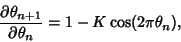(2)

so the circle map is not Area-Preserving. It is related to the Standard Map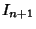(3)(4)

forandcomputed mod 1. Writingas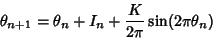(5)

gives the circle map with=and. The unperturbed circle map has the form(6)

Ifis Rational, then it is known as the map Winding Number, defined by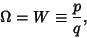(7)

and implies a periodic trajectory, since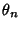will return to the same point (at most) everyOrbits. Ifis Irrational, then the motion is quasiperiodic. Ifis Nonzero, then the motion may be periodic in some finite region surrounding each Rational. This execution of periodic motion in response to an Irrational forcing is known as Mode Locking.

If a plot is made ofvs.with the regions of periodic Mode-Locked parameter space plotted around Rationalvalues (Winding Numbers), then the regions are seen to widen upward from 0 atto some finite width at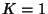. The region surrounding each Rational Number is known as an Arnold Tongue. At, the Arnold Tongues are an isolated set of Measure zero. At, they form a Cantor Set of Dimension. For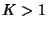, the tongues overlap, and the circle map becomes noninvertible. The circle map has a Feigenbaum Constant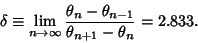(8)